# Lab Notebook

## What is a lab notebook?

A very relevant aspect in the work developed in an experimental science, such as Organic Chemistry, is the annotation of the results obtained. Although the daily laboratory work requires certain skills, however, if the student is not able to organize, analyze and draw the appropriate conclusions from these experiments in a correct way, such work and effort will be useless. Therefore, one of the key tasks that the student must learn as soon as possible is to communicate the results of his experimental work (practical or research) in the form of a laboratory notebook.

This document should be prepared in a clear and concise manner, containing all the experimental work carried out, which will allow the experiments described to be reproduced in the future. In addition, in research, the laboratory notebook plays a relevant role in the possible claim of authorship of a discovery. Research laboratory notebooks (in private companies or academic institutions) are official documents, of a confidential nature, which belong to that institution and are of great help in the subsequent process of publication of patents and scientific articles.

It includes some recommendations and general guidelines necessary for the preparation of a good laboratory notebook and ends with a reminder of the basic calculations that should be included in such a notebook, such as the expression of concentration, the concept of limiting reagent and stoichiometric and yield calculations.

## Notebook structure

It should be clarified that the structure of a laboratory notebook is not exactly the same whether it is a laboratory practical, where the aim is to repeat an already documented chemical recipe, or whether the notebook is intended to describe research experiments. However, both types of laboratory work share many aspects in common. The simplest way to structure a laboratory notebook correctly is to include the following sections:

A few pages at the beginning of the notebook should be set aside for a table of contents, listing the experiments and the pages where they are located. This will make it much easier to find information about a particular experiment in the future.

### Name(s) of person(s) performing

It is important, especially in research, to indicate the author(s) conducting the experiments.

### Date of completion and order number

At the beginning of an experiment, the experiment number, code, etc. as well as the date of the experiment are always noted. So that at all times, the notebook really becomes a laboratory diary.

### Experiment title

It should be clear and descriptive of the experience, for example:

• Synthesis of …………………………….
• Purification of ………………… by recrystallisation …………………….

### Experiment objective

It should be as brief as possible (one or two paragraphs). It will explain the type of experiment or reaction that has been performed (for example: nitration reaction by an electrophilic aromatic substitution process on methyl benzoate), its use in organic chemistry (it is very common, it is very rare, it is used in industry, it is similar to a process that occurs in living beings, etc.) and other general aspects.

### Reaction scheme

A stoichiometrically adjusted (when possible) scheme of the reactions should be prepared. This scheme should include the structural formulas (developed or semi-developed) of all the compounds and chemical reactions involved in the process. It is not advisable to use only the compound names or empirical formulas, as they may lead to confusion, e.g. in case of isomers.

Explanation of the reaction(s): how the reactions take place, whether they are common to other types of substrates, what role each of the reactants plays (acts as oxidant, reductant, nucleophile, base, etc.) and the type of reaction mechanism (SN1, aromatic electrophilic substitution, elimination, etc.). The reaction mechanism should also be explained, with a scheme indicating electron movements by curved arrows and all important reaction intermediates. Key transition states may also be indicated. If applicable, the regiochemistry and stereochemistry of the reaction should be explained and if competitive reactions occur, leading to the formation of side products.

### Identification of reagents and solvents

• Identification (relevant data) of the reagents and products obtained:
• physical properties such as formula, molecular mass, p.f., p.e., density, etc.
• Hazard data and precautions for use (MSDS should be consulted).
• Quantities of reagents and solvents to be used (in grams and moles, and when they are liquids in milliliters). Calculation of the theoretical yield and that obtained for the products.

It is useful to prepare a table in which all the reagents and products are represented, their most relevant characteristics (formula, molecular mass, density if they are liquids, and quantity used in mg, mol and equivalents):

 Compound Mw Density (ml, g, or mg) Amount No. mole … … … … … … … … … … … … … … …

### Procedure and experimental development

The procedure is the method followed to carry out the experiment. It should indicate the set-up (provided it is not too simple), basic operations and techniques used to carry out both the chemical reactions and the isolation and purification of the products, as well as a scheme of separation of the products obtained in the practice. The recipe of the practical manual should not be copied exactly, but the procedure actually followed, since sometimes the practices are repeated with slight modifications.

A brief description of how the experiment was carried out should be given. The actual procedure followed should be described as well as any modifications made to the original procedure, with a brief justification of the reason for the change. First of all the quantities of the products used (in g or mg, ml, mmol and equivalents), thus analyzing the reagents that are used in excess and the limiting reagent(s) that will condition the yield of the reaction.

An important aspect to be taken into account is the inclusion of safety measures to be considered depending not only on the hazardous nature of the reagents and solvents used but also on the basic techniques and operations employed.

Then, the conditions under which the reaction is carried out must be indicated, justifying in a reasoned way the operations that have been performed:

• Solvent and “actual amount” of solvent used.
• Order and manner of addition of reagents.
• Temperature and other conditions used.
• Type of agitation: magnetic, mechanical or manual.
• Equipment used and its configuration: reflux with or without desiccant tower, simple distillation, type of filtration, etc.
• Reaction time.
• Treatment to which the reaction crude is subjected.
Isolation and purification of the products obtained.

It is important to mention any data of interest observed during the course of the experiment, or incidence that occurs such as:

• Colour changes.
• Difficulty in dissolving some reagents.
• Appearance of precipitates.
• Release of heat.
• Generation of gases
• Procedure by which the reaction is monitored: time, aspect ratio, thin layer chromatography, etc.
• Errors detected in the bibliographical description of the practical and how they have been corrected.

Finally, within this section, the amount of product(s) obtained (weight, number of moles and yield), degree of purity, physical appearance (physical state: liquid, amorphous solid, crystalline solid, color, odor, solubility tests, etc.) and its physical and spectroscopic characteristics (melting point, IR and NMR data) if available, assigning the 1H and 13C NMR signals and the most important IR bands, should also be indicated. A common way to present spectroscopic data is in the form of a list or table, or on a drawing of the molecule. It is convenient to paste or attach copies of the spectra obtained (it should also be indicated how the spectra were made, solvent, scale, type of equipment used, etc.). It is also very useful to indicate the chromatographic Rf (indicating the composition of the eluent and type of adsorbent).

When used for the isolation of chromatographic products, the exact procedure must be detailed (e.g. in GC: quantities used, retention times, peak areas, parameters used, types of columns, temperature, etc.).

Thin layer chromatography plates obtained during the experiment can be included, either directly or by means of a photograph or detailed drawing.

### Yield

When applicable, the calculation of the yield of the reaction or sequence of reactions should be made. If a process has been carried out in stages, the yield of each of the reactions should be given, calculating also the overall yield.

Incidents or comments that you consider of interest during the practice.

Indicate whether the reaction took place as expected, whether the pure product could be obtained and whether the spectroscopic and other data are as expected. In case something went wrong, give reasons why.

Explain if there have been any noteworthy incidents.

### Conclusions

State the main conclusions of the experiment: what has been achieved with it and what has been learned. It can also be related to other concepts or reactions studied in the course.

### Bibliography

If bibliographic sources are used, include them here. Note that bibliographic sources should be included in sufficient detail so that the information can be found. When citing a book, not only the authors and title, but also the year, edition and page (AI Vogel, A.R. Tatchell, B.S. Furnis (Author), A.J. Hannaford, P.W. Greig-Smith, A.J. Hannaford, P.W. Greig-Smith, P.W. Greig-Smith Vogel’s Text of Vogel’s Text. Greig-Smith Vogel’s Textbook of Practical Organic Chemistry, Prentice-Hall, 5 Ed. 1989 , pp. 23-34.).

## Some guidelines for the preparation of the notebook

The laboratory notebook should be conceived as a diary in which each and every one of the experiments carried out is recorded with all the incidents of all kinds that have occurred. It must be taken into account that in many occasions it is necessary to repeat an experiment several times, as when a substance is used as raw material in a sequence of reactions and it is necessary to prepare it assiduously or to proceed to the partial modification of a procedure, for example, for the improvement of yields.The general guidelines for the preparation of a good laboratory notebook are as follows:

• The student should become accustomed to writing preferably with ink and not with pencil during the course of the experiment.
• The handwriting and contents must be clear enough so that another person, with our notebook, could reproduce the experiment in the same way, with the same materials and reaching the same results and conclusions.
• Handling loose sheets of paper should be avoided, even if they are to be stapled later. It is much safer to use a notebook with permanently bound sheets and numbered pages.
• It is necessary to write what was actually done in the experiment and not what was supposed to have been done. If we want a successful experiment to be reproducible, it is essential to be faithful to reality. If, on the contrary, the result of our experience is negative, the only way to correct the errors is to have truthful and detailed information.
• It is convenient to write on consecutive sheets (without jumps) and enter the date on which the experiment was performed.
• When developing the procedures, care should be taken to use a concise and clear style, preferably impersonal forms (following the usual style in scientific publications). It is more appropriate to use expressions such as:

a) Product A is dissolved … instead of I dissolved product A …
b) The mixture is separated … instead of To separate the mixture I performed this or that procedure.
c) The yield of the reaction is … instead of I got a yield of …

• The laboratory notebook is a working tool and therefore of frequent use. If additional notes or observations need to be entered, corrections etc. should not be a problem.

For practical purposes, laboratory experiments can be classified into two major groups which require different treatment:

• Synthesis of a product
• Experimental Techniques

## Example of a description of an experiment

The preparation or synthesis of a compound is the obtaining of a substance with the highest possible degree of purity. When describing the synthesis of a product in the laboratory notebook, the following sections should be included:• Title of the experiment. For example: “Synthesis of 1-bromobutane”.
• Scheme with the reaction taking place, noting molecular formula and molecular mass.
• Scheme with the secondary reactions that occur, if these occur and are known.

Reagents and catalysts used including:

• Molecular formula
• Molecular mass
• Physical properties of the reagents: m.p.., density, richness, etc.

Conditions under which the reaction takes place:

• Solvent and quantity of solvent

Form in which the addition of the reagents takes place:

• Mix everything at the beginning
• In small portions if the reagent is solid
• Cold, hot

Temperature at which the reaction takes place (specify it, if it is cold, at room temperature, hot, at the boiling temperature of the solvent.

Reaction time.

Need to carry out the reaction in an inert atmosphere.

Equipment and assemblies used: general purpose or modified.

How to follow the development of the reaction: time, color change, chromatography, spectroscopic techniques.

Methods of isolation and purification of the product(s) obtained.

Quantity of final product and calculation of yield in %.

Physical properties of pure products:

• Melting point, m.p. or boiling point, b.p.
• Rotational power if it is a chiral molecule

Spectroscopic data obtained from IR and NMR 13C, 1H and UV/Vis spectra.

Observed stability of the product and storage conditions.

Some of the data collected in the notebook can be given in tabular form.

## Basic calculations

### Solutions

To calculate the concentration of a given solution we must know the amount of solute and solvent or solution in the solution. Depending on the units in which the components of the solution are defined, we can express this concentration in different ways:

• Weight percentage: Number of grams of solute divided by 100 grams of solution.
• Parts per million (ppm): Obtained by dividing the mass of a component by the total mass of the solution (106).
• Parts per billion (ppb): This is obtained by dividing the mass of a component by the mass of the solution (109). (In this definition, 109 is considered instead of 1012 as would correspond to the English meaning of billion, a million million million. This is so because in American English, the word billion means a thousand times a million).
• Percentage volume: This is the cm3 of solute divided by 100 cm3 of solution.
• Grams/liter: Grams of solute divided by liter of solution. Grams per liter indicates the mass of solute, expressed in grams, contained in a given volume of solution (not solvent), expressed in liters.
• Molarity, M: Number of moles of solute divided by liter of solution. The molarity is the amount of solute (moles) contained in a certain volume of solution (liters), i.e. M = m/V. The number of moles of solute equals the ratio between the mass of solute and the mass of one mole (molar mass) of solute.
• Normality, N: Number of solute equivalents divided by liter of solution.

Where the number of equivalents = mass of solute divided by the equivalent mass, and the equivalent mass = mass of molecules divided by the number of H+, HO in acid-base reactions and in redox reactions would be the number of electrons exchanged.

• Molality, m: Number of moles of solute divided by kg of solvent.
• Molar fraction, X: The mole fraction of a component in a solution is defined as the ratio of the moles of that component to the total moles of the substances in the solution.

### Stoichiometry and Balancing Reactions

Stoichiometry measures the mass ratios of the chemical elements involved in a chemical reaction.

Transformations (chemical reactions) are rearrangements of atoms in molecules and are therefore governed by the law of conservation of mass (atoms are neither created nor destroyed during a chemical reaction). Therefore, there must be the same number of atoms before (reactants) and after (products) the reaction.

For all these reasons, in a chemical equation the same number of atoms of each element must appear on both sides of the arrow. If this is so, the equation is said to be adjusted.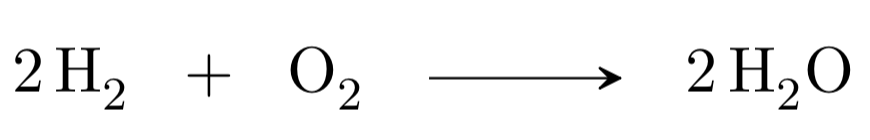Reagents = 4H and 2O

Products = 4H and 2O

The steps to follow to adjust a chemical reaction are as follows:

• Determine which are the reactants and products.
• Write an unadjusted equation using the formulas of the reactants and products, checking that no atoms are missing.
• To adjust the reaction determining the stoichiometric coefficients for each molecule of reactant/s and product/s that give us equal number of each type of atom in the reaction. Whenever possible we should express the stoichiometric coefficients as integers.

For example, the methane combustion reaction gives carbon dioxide and water. Therefore, we first write the unadjusted equation: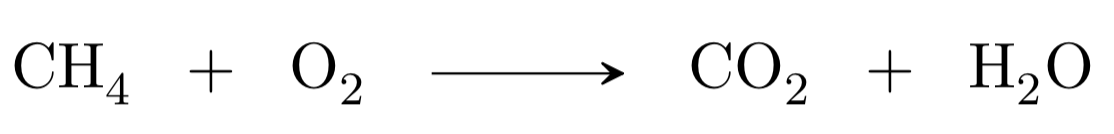The second step would be to adjust the atoms in reactants and products, we begin with carbon, as there is one in the reactants and another in the products, it would be adjusted. We continue with hydrogen, there are four in the reactants (methane) and only two in the products (water), therefore we must multiply by two the water: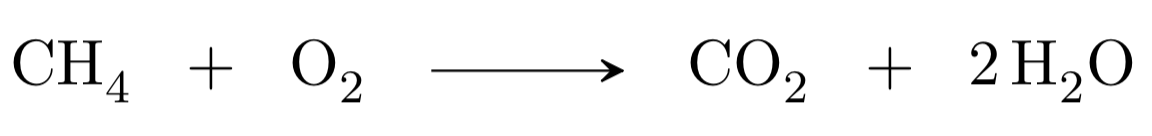We finish with the oxygen, we have two atoms in the reactants and four atoms in the products, therefore, if we put as stoichiometric coefficient of the oxygen of the reactants a two it will be adjusted: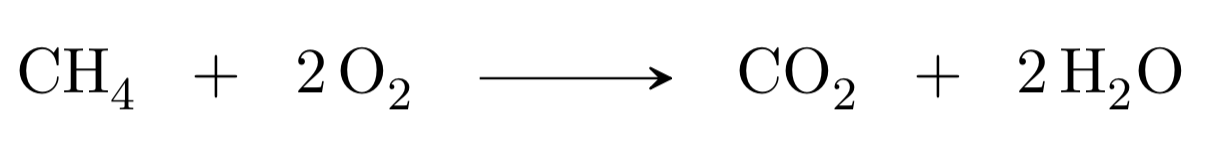We check once again that the numbers of atoms are the same in reactants and products:

Reagents: 1C + 4H + 4O

Products: 1C + 4H + 4O

### Limiting reagent

It is the molecule in a chemical reaction (either reactant or product) that determines the amount of product that will be formed in that chemical transformation. It is used, in an adjusted reaction, to know the moles of a product that are obtained from a given number of moles of a reactant.
This is because when we carry out a reaction in an experiment or practical, the reactants that we weigh and measure are not exactly in the stoichiometric quantities of a given reaction. Sometimes, excesses of some reagents are used, therefore, some of the reagents are totally consumed in the reaction while others are left over at the end of the reaction. The reagent that is consumed first (stopping the reaction) is called the limiting reagent, since the amount we put in determines the total amount of products formed.

The limiting reagent allows the most expensive reagent to be completely consumed in a chemical reaction.

### Yield of a reaction

The absolute yield of a chemical reaction is the amount of pure, dry product obtained in a reaction. It is usually expressed as relative or percentage yield (%) and serves as a measure of the efficiency of a chemical reaction in synthesis.

Before calculating the yield of a reaction (necessary at the time of elaborating the laboratory notebook) it will be necessary to know the stoichiometry (the adjustment of the chemical reaction so that there is the same number and type of atoms in the reactants and in the products) that presents the reaction. In addition, we will have to know which is the limiting reagent (the one that expressed in mol is in a smaller quantity according to the stoichiometry). We can find two situations when calculating the yield of a chemical process.

• Yield of a simple reaction: it is expressed as relative yield (in percent, %), and results from dividing the moles of product obtained by the theoretical moles of product (maximum amount of product that would be obtained if the entire amount of limiting reagent reacted).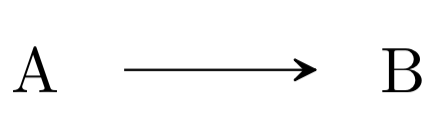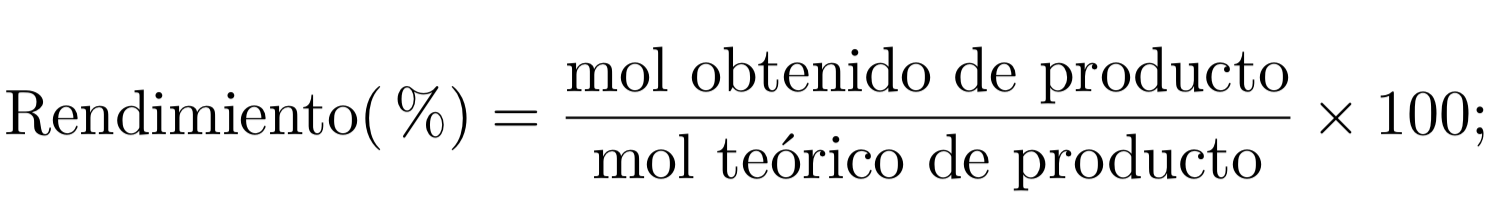• Yield of a multistep reaction: the overall performance of a transformation that takes place with the many chemical reactions are calculated by multiplying the partial yields (expressed as a percentage of one) of each of the reactions that comprise it.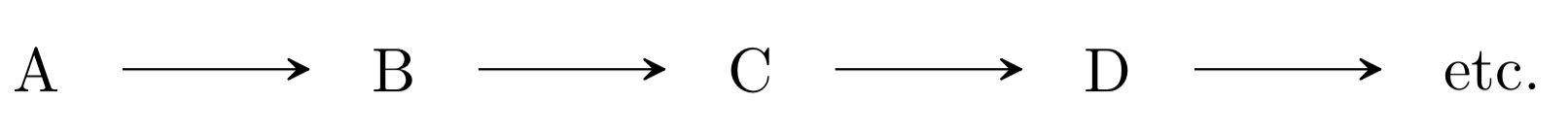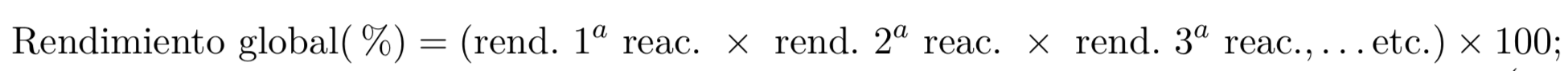Yield of a transformation consisting of several reactions: the overall yield of a transformation involving several chemical reactions is calculated by multiplying the partial yields (expressed as a percentage of one) of each of the reactions involved.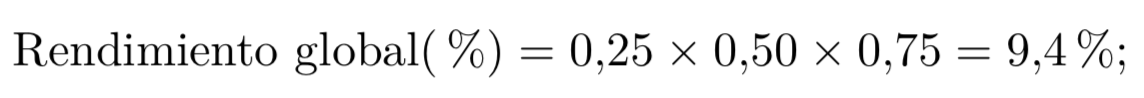Shares